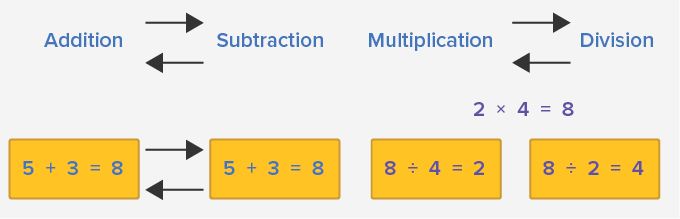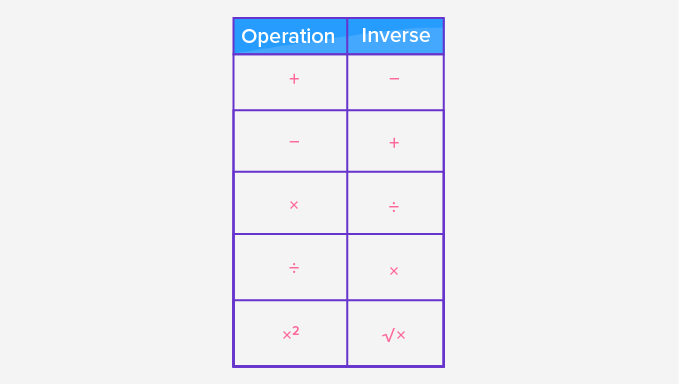Inverse Operations - Definition with Examples

The Complete K-5 Math Learning Program Built for Your Child

• 30 Million Kids

Loved by kids and parent worldwide

• 50,000 Schools

Trusted by teachers across schools

• Comprehensive Curriculum

Aligned to Common Core

Inverse Operations

Operation is a mathematical process involving addition, subtraction, multiplication, division, squaring, square roots, etc.

All the given symbols (+, −, ×, ÷) in mathematics are known as operators.

An inverse operation reverses the effect of the first operation.

For example, if we operated adding two numbers say 5+3 = 8

The inverse operation of this would be the subtraction of these two numbers: 5-3= 2.Inverse Operators

The primary operations in mathematics are addition, subtraction, multiplication, and division.  The inverse operations of these are given in the table below:

Inverse operations

 Operations Inverse operations Addition Subtraction Subtraction Addition Multiplication Division Division Multiplication

Inverse operatorsMore examples of inverse operations

 Operator + _ × ÷ Inverse operator _ + ÷ × Example 5+4 = 9  5-4 =1 6-3 = 3 6+3 =9 2 × 3 =6  6 ÷ 3 = 2 8÷ 2 = 4 2 × 4 = 8

Properties of Inverse Operations

Inverse additive property: The inverse operation of the addition of two same numbers will result in a zero.

2 + (-2) = 0

or

When we add a number to its opposite, the result is always 0.

Inverse multiplicative property: When we multiply a number is by its (multiplicative) inverse, the result is always 1.

Multiplicative inverse of 10 is 110

So, 10 × 110=1

Additive Property: Consider the operation , 5 + 0 = 5

The inverse operation is,  5-  0  = 5

Thus, when we add any number to zero, both the operation and inverse operation will give the same number.

Multiplicative Property: Consider the operation,  5 × 1 = 5

The inverse operation is, 5 ÷ 1 = 5

Thus, when we multiply any number with 1, both the operation and inverse operation will give the same number.

Won Numerous Awards & Honors